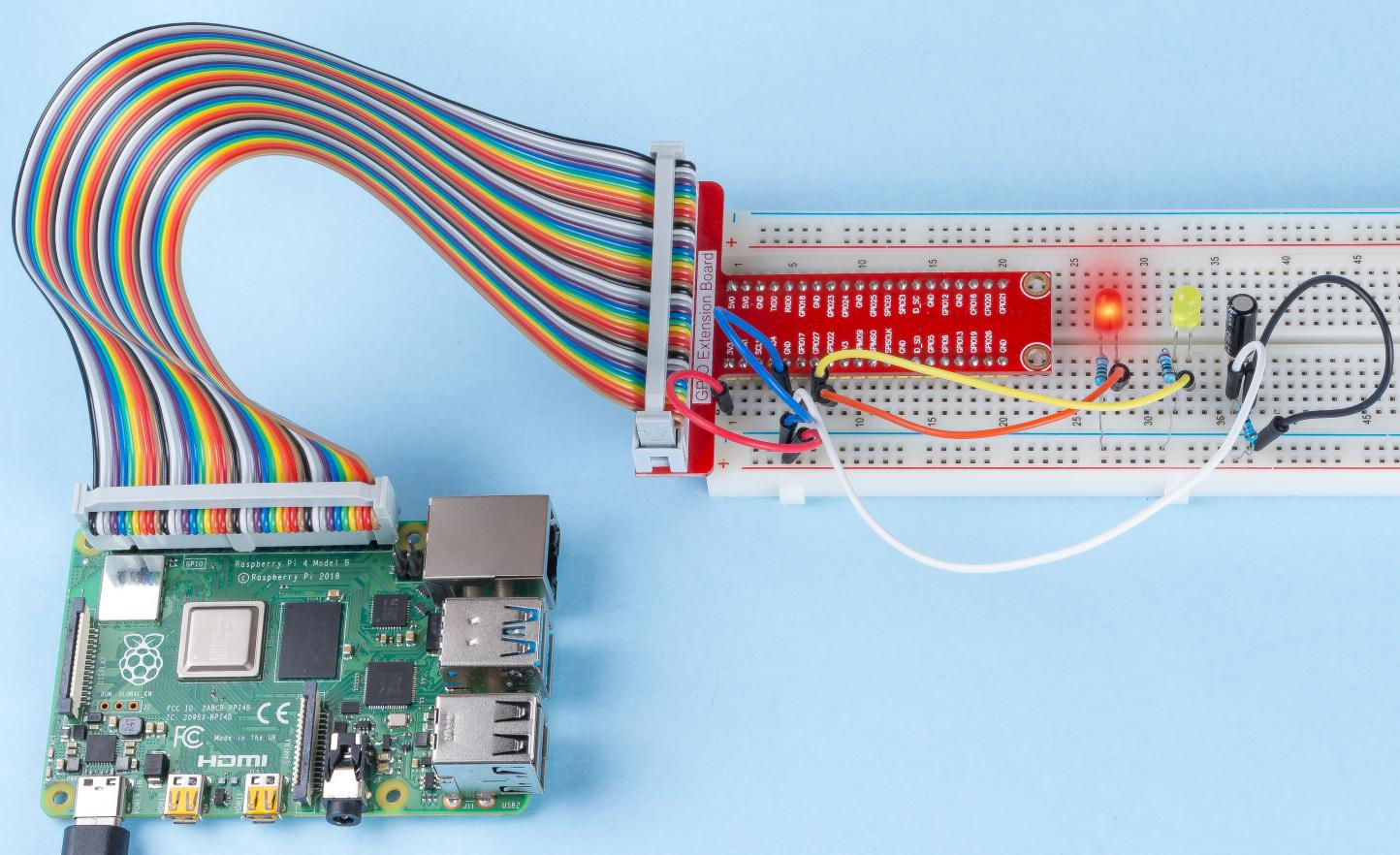# 2.1.3 Tilt Switch¶

## Introduction¶

This is a ball tilt-switch with a metal ball inside. It is used to detect inclinations of a small angle.

## Components¶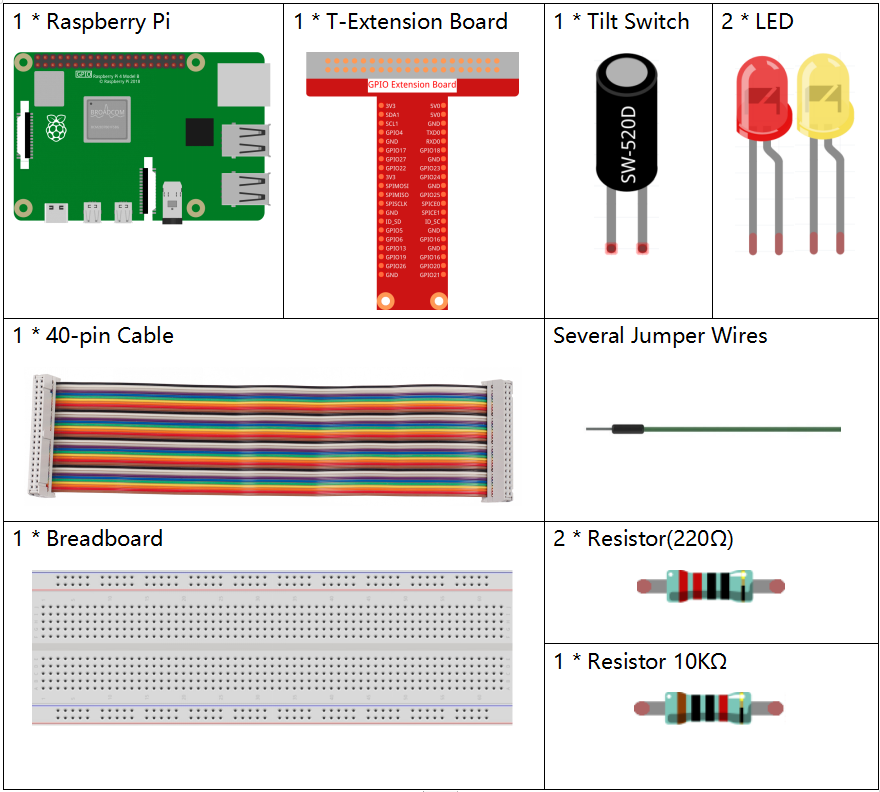## Principle¶

Tilt

The principle is very simple. When the switch is tilted in a certain angle, the ball inside rolls down and touches the two contacts connected to the pins outside, thus triggering circuits. Otherwise the ball will stay away from the contacts, thus breaking the circuits.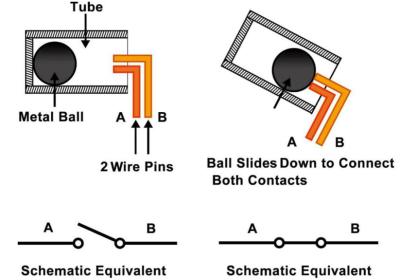## Schematic Diagram¶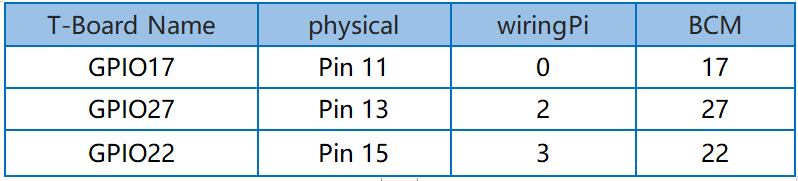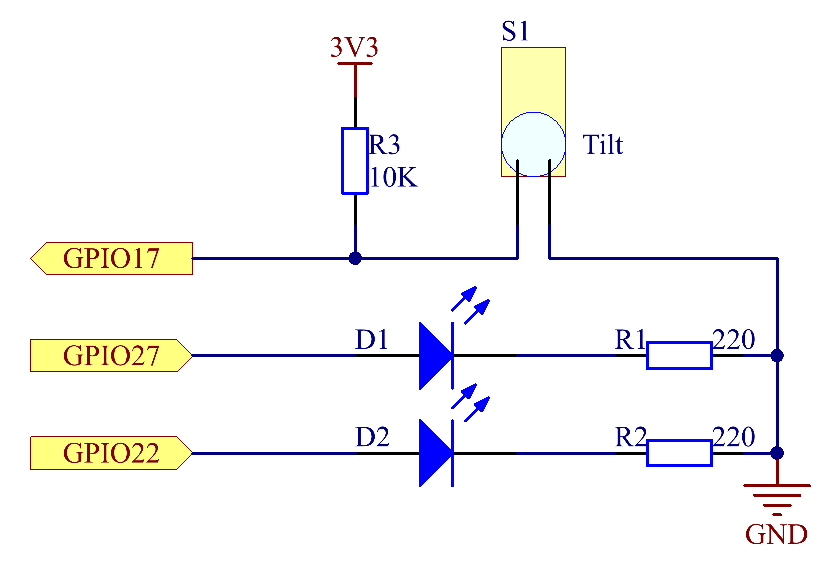## Experimental Procedures¶

Step 1: Build the circuit.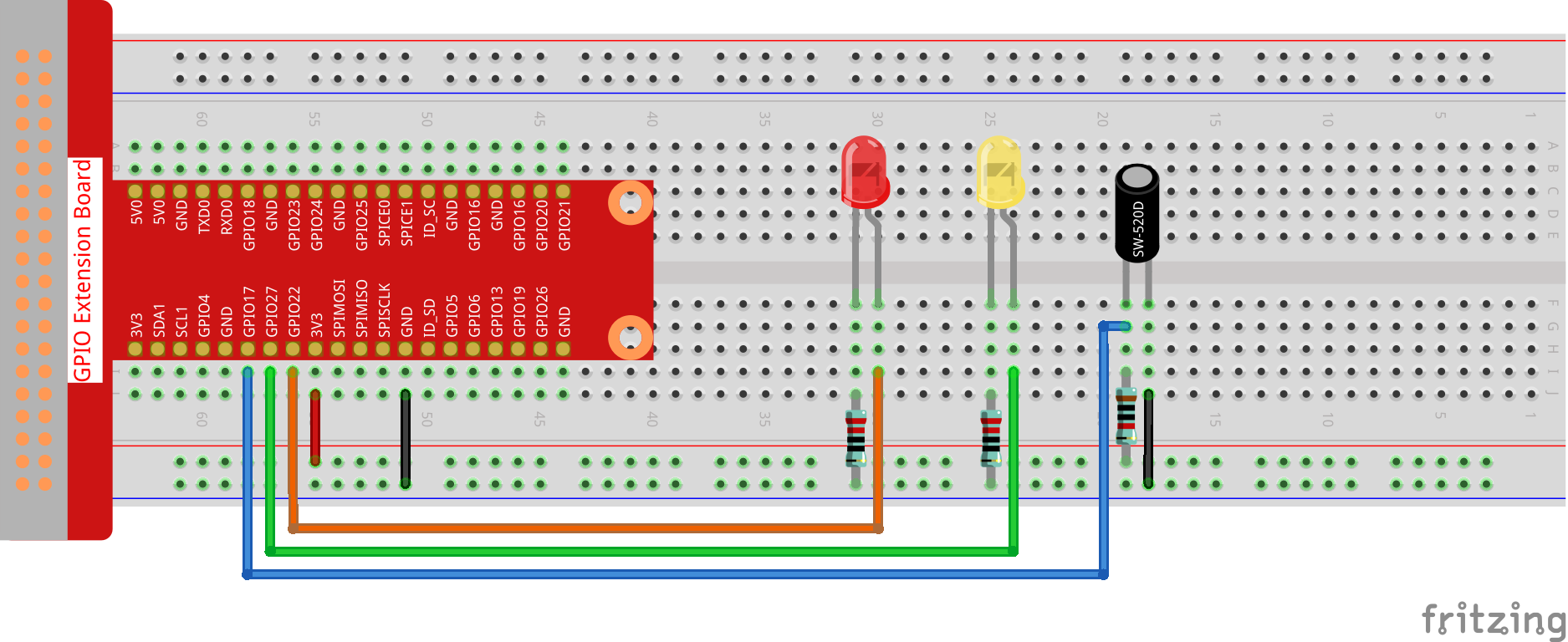### For C Language Users¶

Step 2: Change directory.

```cd ~/davinci-kit-for-raspberry-pi/c/2.1.3/
```

Step 3: Compile.

```gcc 2.1.3_Tilt.c -lwiringPi
```

Step 4: Run.

```sudo ./a.out
```

Place the tilt horizontally, and the green LED will turns on. If you tilt it, "Tilt!" will be printed on the screen and the red LED will lights on. Place it horizontally again, and the green LED will turns on again.

Note

If it does not work after running, or there is an error prompt: "wiringPi.h: No such file or directory", please refer to C code is not working?.

Code

```#include <wiringPi.h>
#include <stdio.h>

#define TiltPin     0
#define Gpin        2
#define Rpin        3

void LED(char* color)
{
pinMode(Gpin, OUTPUT);
pinMode(Rpin, OUTPUT);
if (color == "RED")
{
digitalWrite(Rpin, HIGH);
digitalWrite(Gpin, LOW);
}
else if (color == "GREEN")
{
digitalWrite(Rpin, LOW);
digitalWrite(Gpin, HIGH);
}
else
printf("LED Error");
}

int main(void)
{
if(wiringPiSetup() == -1){ //when initialize wiring failed,print message to screen
printf("setup wiringPi failed !");
return 1;
}

pinMode(TiltPin, INPUT);
LED("GREEN");

while(1){
delay(10);
LED("RED");
printf("Tilt!\n");
delay(100);
}
}
delay(10);
LED("GREEN");
}
}
}
return 0;
}
```

Code Explanation

```void LED(char* color)
{
pinMode(Gpin, OUTPUT);
pinMode(Rpin, OUTPUT);
if (color == "RED")
{
digitalWrite(Rpin, HIGH);
digitalWrite(Gpin, LOW);
}
else if (color == "GREEN")
{
digitalWrite(Rpin, LOW);
digitalWrite(Gpin, HIGH);
}
else
printf("LED Error");
}
```

Define a function LED() to turn the two LEDs on or off. If the parameter color is RED, the red LED lights up; similarly, if the parameter color is GREEN, the green LED will turns on.

```while(1){
delay(10);
LED("RED");
printf("Tilt!\n");
}
}
delay(10);
LED("GREEN");
}
}
}
```

If the read value of tilt switch is 0, it means that the tilt switch is tilted then you write the parameter "RED" into function LED to get the red LED lighten up; otherwise, the green LED will lit.

### For Python Language Users¶

Step 2: Change directory.

```cd ~/davinci-kit-for-raspberry-pi/python/
```

Step 3: Run.

```sudo python3 2.1.3_Tilt.py
```

Place the tilt horizontally, and the green LED will turns on. If you tilt it, "Tilt!" will be printed on the screen and the red LED will turns on. Place it horizontally again, and the green LED will lights on.

Code

Note

You can Modify/Reset/Copy/Run/Stop the code below. But before that, you need to go to source code path like `davinci-kit-for-raspberry-pi/python`.

```import RPi.GPIO as GPIO
import time

dhtPin = 17

GPIO.setmode(GPIO.BCM)

MAX_UNCHANGE_COUNT = 100

STATE_INIT_PULL_DOWN = 1
STATE_INIT_PULL_UP = 2
STATE_DATA_FIRST_PULL_DOWN = 3
STATE_DATA_PULL_UP = 4
STATE_DATA_PULL_DOWN = 5

GPIO.setup(dhtPin, GPIO.OUT)
GPIO.output(dhtPin, GPIO.HIGH)
time.sleep(0.05)
GPIO.output(dhtPin, GPIO.LOW)
time.sleep(0.02)
GPIO.setup(dhtPin, GPIO.IN, GPIO.PUD_UP)

unchanged_count = 0
last = -1
data = []
while True:
current = GPIO.input(dhtPin)
data.append(current)
if last != current:
unchanged_count = 0
last = current
else:
unchanged_count += 1
if unchanged_count > MAX_UNCHANGE_COUNT:
break

state = STATE_INIT_PULL_DOWN

lengths = []
current_length = 0

for current in data:
current_length += 1

if state == STATE_INIT_PULL_DOWN:
if current == GPIO.LOW:
state = STATE_INIT_PULL_UP
else:
continue
if state == STATE_INIT_PULL_UP:
if current == GPIO.HIGH:
state = STATE_DATA_FIRST_PULL_DOWN
else:
continue
if state == STATE_DATA_FIRST_PULL_DOWN:
if current == GPIO.LOW:
state = STATE_DATA_PULL_UP
else:
continue
if state == STATE_DATA_PULL_UP:
if current == GPIO.HIGH:
current_length = 0
state = STATE_DATA_PULL_DOWN
else:
continue
if state == STATE_DATA_PULL_DOWN:
if current == GPIO.LOW:
lengths.append(current_length)
state = STATE_DATA_PULL_UP
else:
continue
if len(lengths) != 40:
#print ("Data not good, skip")
return False

shortest_pull_up = min(lengths)
longest_pull_up = max(lengths)
halfway = (longest_pull_up + shortest_pull_up) / 2
bits = []
the_bytes = []
byte = 0

for length in lengths:
bit = 0
if length > halfway:
bit = 1
bits.append(bit)
#print ("bits: %s, length: %d" % (bits, len(bits)))
for i in range(0, len(bits)):
byte = byte << 1
if (bits[i]):
byte = byte | 1
else:
byte = byte | 0
if ((i + 1) % 8 == 0):
the_bytes.append(byte)
byte = 0
#print (the_bytes)
checksum = (the_bytes + the_bytes + the_bytes + the_bytes) & 0xFF
if the_bytes != checksum:
#print ("Data not good, skip")
return False

return the_bytes, the_bytes

def main():

while True:
if result:
humidity, temperature = result
print ("humidity: %s %%,  Temperature: %s C`" % (humidity, temperature))
time.sleep(1)

def destroy():
GPIO.cleanup()

if __name__ == '__main__':
try:
main()
except KeyboardInterrupt:
destroy()
```

Code Explanation

```GPIO.add_event_detect(TiltPin, GPIO.BOTH, callback=detect, bouncetime=200)
```

Set up a detect on TiltPin, and callback function to detect.

```def Led(x):
if x == 0:
GPIO.output(Rpin, 1)
GPIO.output(Gpin, 0)
if x == 1:
GPIO.output(Rpin, 0)
GPIO.output(Gpin, 1)
```

Define a function Led() to turn the two LEDs on or off. If x=0, the red LED lights up; otherwise, the green LED will be lit.

```def Print(x):
if x == 0:
print ('    *************')
print ('    *   Tilt!   *')
print ('    *************')
```

Create a function, Print() to print the characters above on the screen.

```def detect(chn):
Led(GPIO.input(TiltPin))
Print(GPIO.input(TiltPin))
```

Define a callback function for tilt callback. Get the read value of the tilt switch then the function Led（） controls the turning on or off of the two LEDs that is depended on the read value of the tilt switch.

## Phenomenon Picture¶